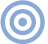Reference

Audio Basics: A Is For Ampere Page 3

Controlling Current Flow
While sticking a wire across a battery makes for an enlightening illustration, it isn't really all that good an idea. Wire is such a good conductor that it will pass as many electrons as the battery can supply. In fact, it will pass so many that friction between them will cause the wire to heat up, and if the wire is thin enough, it will melt. If it's too thick to heat, it will simply "short-circuit" the battery, so that no potential difference (voltage) can exist between the battery terminals, in which case the battery will become extremely agitated, heat up, and possibly even explode, wiping out civilization as we knew it. Either way, it's destruction---for the wire or the battery. For a controlled current flow, we need to limit the current-passing capability of the circuit, and to do this we use (Fanfare!) a resistor (fig.2).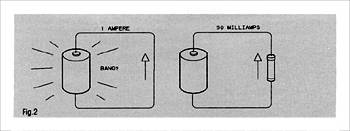Fig.2 A heavy wire across a battery will cause it to deliver as much current as it can. A resistor in the circuit will limit current flow according to its resistance; the higher the resistance, the less the current.

A resistor, which is often nothing more than a thin film of carbon or metal on a ceramic former, provides fewer electron-conducting paths through it than a wire, so that, for a given applied EMF, current flow through the circuit is reduced. Thus, we can stick it in series with the wire across our battery and get a moderate current flow that will not burn out the wire or blow up the battery. It will merely waste energy. Actually, wires have some resistance too, and the thinner the wire the higher its resistance per foot (or inch, or whatever). For our purposes, the important differences between a resistor and a thin wire with resistance are that 1) a resistor is fatter and thus has more surface area from which to dissipate frictional heat, and 2) a resistor has a known value of resistance, which is marked right on it, either as a number or a color code.

The unit of resistance is the ohm, which you should remember. You will probably never need to decipher the color code, (footnote 5) but just in case, here it is: Black=0, Brown=1, Red=2, Orange=3, Yellow=4, Green=5, Blue=6, Violet=7, Gray=8, and White=9. There are usually three colored stripes side by side: The first two are number digits, the third is a decimal multiplier. That is, a red third-stripe would multiply the first two digits by 100. A resistor marked red-red-brown would have a value of 2 (red) 2 (red) times 10 (brown) = 220 ohms.

A fourth band, usually at the opposite end of the resistor to the value code, indicates tolerance, with silver implying ±10%, gold ±5%, red ±2%, and brown ±1%. A silver band on our 220 ohm resistor implies that its real value lies between 198 and 242 ohms, which is close enough for many purposes, though not for high-end audio!

Resistors are also sold in different physical sizes, for specified amounts of heat (power) dissipation ranging from 1/10W to several hundred watts. It is customary to use the smallest you can get away with, otherwise a transistor radio would be the size of a refrigerator.

Apart from limiting current flow, resistors have another useful function: They can provide accurate fractional division of an electrical voltage. In fig.3, two resistors of identical value are connected in series across a 10V battery (footnote 6). It seems obvious that the junction between them will be halfway between that, or 5V, so by connecting to point A and point B of the resistor network, we can draw off precisely half of the source voltage. If resistor R2 is 1/3 the total value of both resistors, the voltage between A and B will be 1/3 the source voltage. Et cetera. If, instead of two fixed resistors, we use a single one with an adjustable slider (fig.4), we can select any dividing fraction of the source voltage that we desire. This is how a volume control works. It is also how amplification works, but we'll get to that later.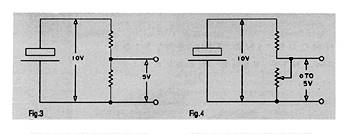Fig.3 Two identical resistors across a voltage source (zigzag lines) will divide the total available voltage in half at their junction.

Fig. 4 A variable resistor (bottom) equal to the fixed one in fig. 2 provides a choice of voltages between zero and half the supply voltage. Eliminating the top resistor would allow you to select anything from 0 to 10V.

Footnote 5: If you do want to remember this code, just remember a mnemonic I was reminded of by George Graves: "Busy (Black-0) Boys (Brown-1) Rest (Red-2) On (Orange-3) Yale (Yellow-4) Ground (Green-5) But (Blue-6) Vassar (Violet-7) Girls (Grey-8) Work (White-9)." Again, "Busy boys rest on Yale ground, but Vassar girls work."

Footnote 6: Okay, purists, I know a single battery cell can't deliver 10V, so think of it as six cells delivering almost 10V.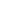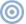ARTICLE CONTENTSX